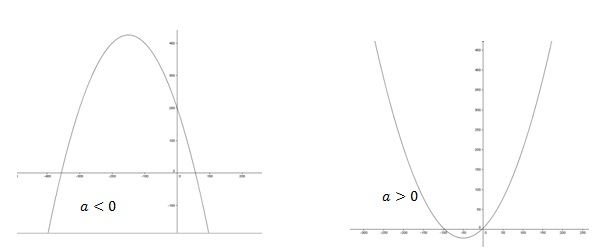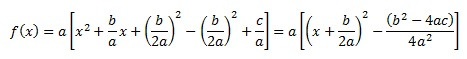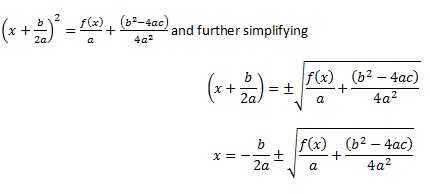# How to find the Axis of Symmetry of a Quadratic Function

## What is a Quadratic Function

A polynomial function of second degree is called a quadratic function. Formally,  f(x)=ax2+bx+c is a quadratic function, where a,b and c are real constant and a≠0 for all values of x. The graph of a quadratic function is a parabola.## How to find the axis of symmetry of a Quadratic Function

Any quadratic function shows lateral symmetry across y axis or a line parallel to it. The axis of symmetry of a quadratic function can be found as follows:

f(x)=ax2+bx+c where a,b,c,x∈R and a≠0

Writing x terms as a full square we have,By rearranging the terms of the above equationThis implies that, for every possible value f(x) there are two corresponding x values. This can be clearly seen in the diagram below.

distance to the left and right of the value -b/2a. In other words value -b/2a is always the midpoint of a line joining the corresponding x values (points) for any given f(x).

Therefore ,
x=-b/2a is the equation of the axis of symmetry for a given quadratic function in the form f(x)=ax2+bx+c

## How to find the axis of symmetry of a Quadratic Function – Examples

• A quadratic function is given by f(x)=4x2+x+1. Find the symmetric axis.

x=-b/2a=-1/(2×4)=-1/8

Therefore, equation of the axis of symmetry is x=-1/8

• A quadratic function is given by expression f(x)=(x-2)(2x-5)

By simplifying the expression we have f(x)=2x2-5x-4x+10=2x2-9x+10

We can deduce that a=2 and b=-9. Therefore, we can get the axis of symmetry as

x=- (-9)/(2×2)=9/4